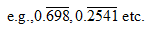# SBI PO Prelims Exam 2015: Quantitative Aptitude: Concept & Sample Questions: Fractions & Decimal

The banking team of jagranjosh.com has come up with concept and sample questions for Fractions and Decimal. The concept provided by us will help you to understand the topic. The sample questions offered by us are framed by keeping in view need of the question paper.

May 6, 2015 18:00 IST

The banking team of jagranjosh.com has come up with concept and sample questions for Fractions and Decimal. The concept provided by us will help you to understand the topic. The sample questions offered by us are framed by keeping in view need of the question paper.

What is a Fraction?

When a unit is divided into any number of equal parts, then one or more of these parts is termed as a fraction of the unit.

e. g., If 1 is to be divided into two equal parts, then 1 is divided by 2 and it is expressed as 1/2.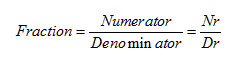Properties of Fraction

1. If numerator = denominator, then the value of fraction is equal to 1.

2. When numerator = 0 while denominator ≠ 0, then fraction = 0.

3. The denominator of a fraction is always assured to be non-zero but in case when denominator = 0, then fraction = (infinity).

4. When numerator and denominator are multiplied or divided by the same number, then value of fraction remains unchanged.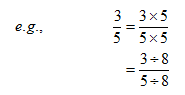5. If numerator and denominator have no common factor, then the fraction is said to be in its lowest terms.

6. A fraction is a rational number.

Operations on Fractions

1. Addition and Subtraction of Decimal Fraction

To add or subtract decimal fractions, the given numbers are so written under each other that the decimal points lie in one column and the numbers so arranged can now be added or subtracted as per the conventional method of addition and subtraction.

Exp 1. Solve the following expressions.

(i) 453.625 + 0.01 + 2.43 =?

Sol. (i) 453.625

0.010

+2.430

456.065

2. Multiplication of Two or More Decimal Fractions

Given fractions are multiplied without considering the decimal points and then in the product, decimal point is marked as many places of decimal as the sum of the numbers of decimal places in the given numbers.

Exp 2. Solve the following expressions.
(i) 43 x 0.13 =?

Sol. (i) 43 x 1 3 = 599

Sum of decimal places = 1 + 2 = 3

Required product = 0.599

3. Multiplication of Decimal Fraction by an Integer

Given integer is multiplied by the fraction without considering the decimal point and then
in the product decimal is put as many as places before as that in the given decimal fraction.

Exp.3 Find the value of
(i) 19.72 x 4

Sol. (i) 19.72 x 4

Multiplying without taking decimal point into

Consideration 1972 x 4 = 7888

So, 19.72 x 4 = 78.88

(In the given decimal fraction, decimal point is two places before, so in the product decimal
point will also be put two places before.)
Similarly,

4. Dividing a Decimal Fraction by an Integer

Do simple divisions i.e., divide the given decimal number without considering the decimal, by
given counting number and in the quotient so obtained, place the decimal point as many places
of decimal as what are there in the dividend.

Exp 4. Divide (i) 0.81 by 9,

(ii) 1.2875 by 25 and

(iii) 0.00049 by 7.

Sol. (i)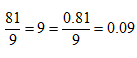(Two places of decimal)

Division of Decimal Fractions

In such divisions, dividend and divisor both are multiplied first by a suitable multiple of 10 to
convert divisor into a whole number and then above mentioned rule of division is followed.

Exp 5. Divide (i) 42 by 0.007 and

Sol. (i)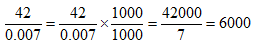Recurring Decimals

The decimal fractions, in which one or more decimal digits are repeated again and again, are
called recurring decimal fractions.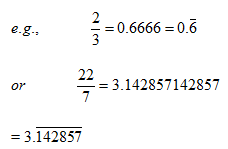Here, a line is drawn on the digits which are repeated.

Pure Recurring Decimal Fractions

When all the figures in a decimal fraction are repeated after the decimal point, then the
decimal fraction is called a pure recurring decimal.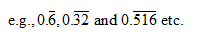Mixed Recurring Decimal Fractions

A decimal fraction, in which some figures are not repeated while some of them are repeated, is
called a mixed recurring decimal.